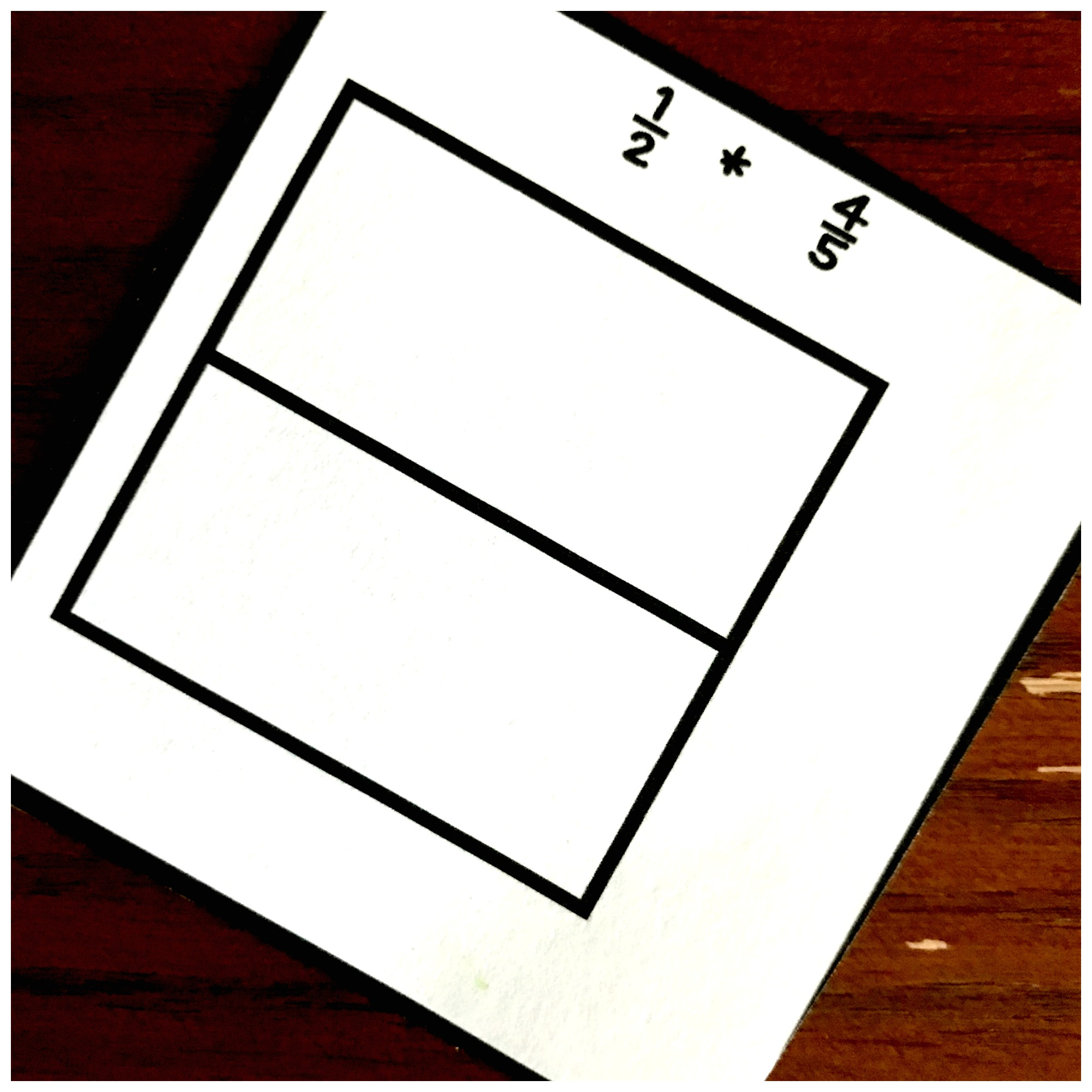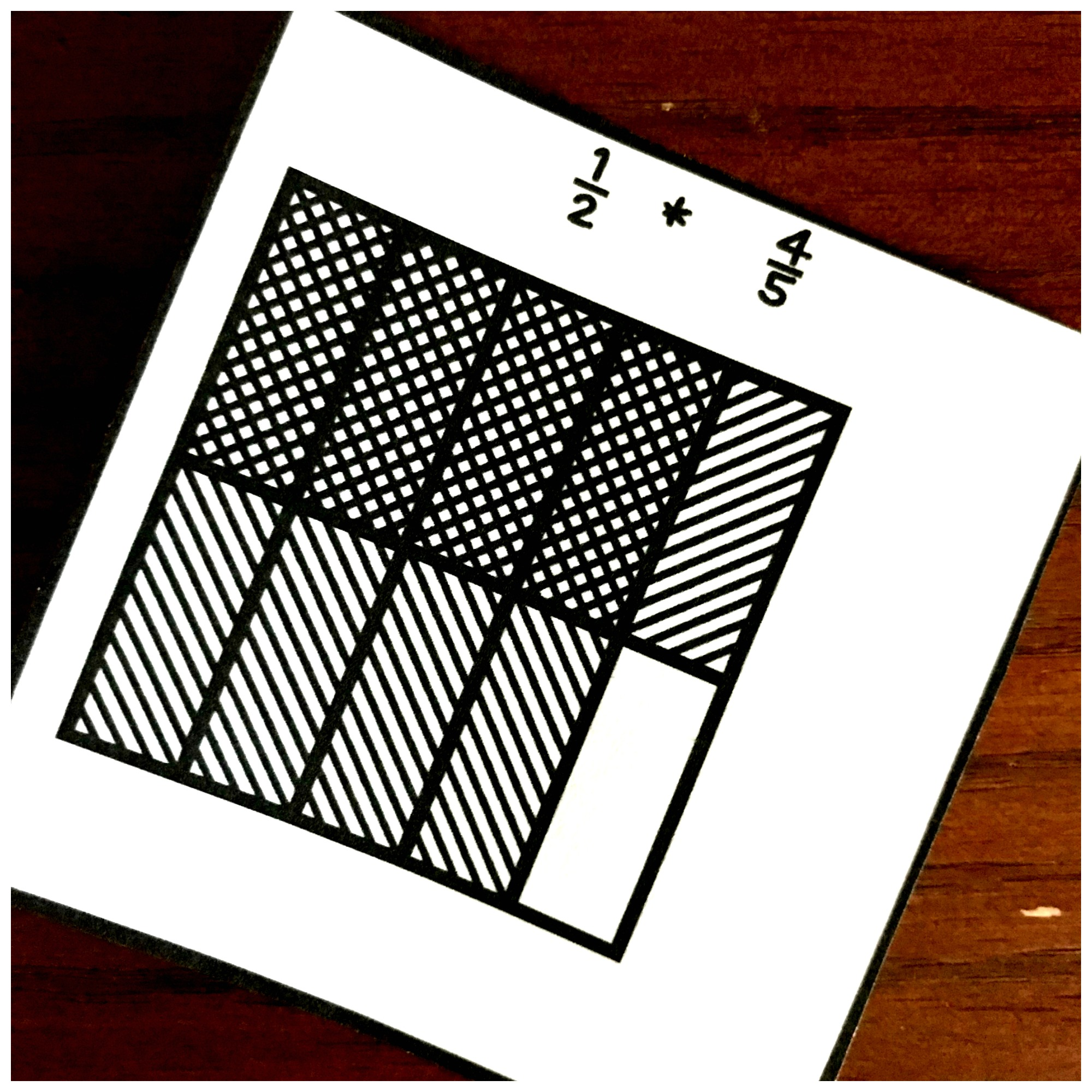Home » Math » How to Multiply Fractions by Fractions – Step by Step Instructions with Free Printable

How to Multiply Fractions by Fractions – Step by Step Instructions with Free Printable

This interactive notebook will walk you and your children through how to Multiplying Fractions with Models. A free printable is included!I have to admit when I taught this concept years ago…..I struggled with it. My special ed kids struggled drawing boxes and then seeing the shaded parts.

Multiplying fractions with models is difficult, but it is important.

If you have been on my blog you know my philosophy. Children need to understand what they are doing in math, not just plug numbers into a formula……and using models is a wonderful way to do this. Even if it is difficult.

So today is all about introducing multiplying fractions by fractions with a model.

Looking for multiplying fractions by a whole number? This may help!Or If you need mixed number multiplication try this!Prep-Work for Multiplying Fractions With Models Interactive Notebook

So there is very little prep-work with this activity.

1. Print of pages
2. Provide colored pencils, pencils, and a math notebook.

And you are ready to go.

What’s Next?

Now comes the fun part…..helping children understand multiplying fractions with models.

Step 1

First, draw a rectangle. Then, look at the first fraction in the expression and divide the height of the rectangle by the denominator.Step 2

Use the numerator to determine how many parts that need to be shaded in.Step 3

Next, we divide the width of the rectangle up by using the number in the denominator of the second fraction.Step 4

Now we use the numerator to determine how many parts that need to be shaded in. I recommend using a different pattern or color to shade in the second fraction!Step 5

Finally, we count up all the parts that have been shaded twice. This is the numerator.

Count up how many parts are in the whole rectangle and that is the denominator.That’s it!!! That’s all you have to do to teach multiplying fractions with models. And now it is time for some practice.

This activity may help your children get it some extra practice in a fun, hands-on way!Or check out our 100 Fraction Activities!Want to get all my multiplying fractions by fractions activities in on place. Get them here!!

You’ve Got This

Rachel